# 5 Polygons

Given k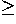3 points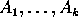in the plane, in a certain order, we obtain a k-sided polygon or k-gon by connecting each point to the next, and the last to the first, with a line segment. The points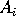are the vertices and the segments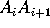are the sides or edges of the polygon. When k=3 we have a triangle, when k=4 we have a quadrangle or quadrilateral, and so on (see table of regular polygons). Here we will assume that all polygons are simple: this means that no consecutive edges are on the same line, and no two edges intersect (except that consecutive edges intersect at the common vertex). See Figure 1.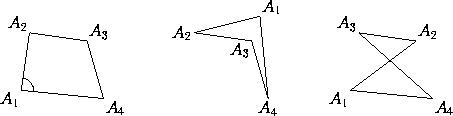Figure 1: Two simple quadrilaterals (left and middle) and one that is not simple (right). We will not consider non-simple polygons.

When we refer to the angle at a vertex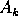we have in mind the interior angle (as marked in the leftmost polygon in Figure 1). We denote this angle by the same symbol as the vertex. The complement ofis the exterior angle at that vertex; geometrically, it is the angle between one side and the extension of the adjacent side. In any k-gon, the sum of the angles equals 2(k-2) right angles, or 2(k-2)×90°: for example, the sum of the angles of a triangle is 180°.

The area of a polygon whose verticeshave coordinates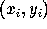, for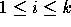, is the absolute value of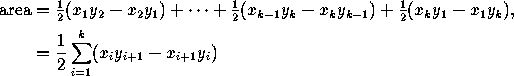where in the sumation we take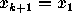and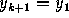. In particular, for a triangle we have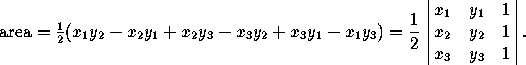In oblique coordinates with angle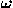between the axes, the area is as given above, times sin.

If the vertices have polar coordinates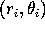, for, the area is the absolute value of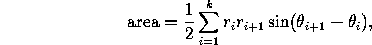where we take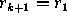and.

Formulas for specific polygons in terms of side lengths, angles, etc. are given in the next sections.

Next: 5.1 Triangles
Up: Part I: Two-Dimensional Geometry
Previous: 4.3 Concurrence and CollinearityThe Geometry Center Home Page

Silvio Levy
Wed Oct 4 16:41:25 PDT 1995

This document is excerpted from the 30th Edition of the CRC Standard Mathematical Tables and Formulas (CRC Press). Unauthorized duplication is forbidden.